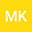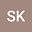Numerical Calculations of the MEW Equation from a New Perspective
••• Melike Karta,
• Selcuk KUTLUAY
Melike Karta
Agri Ibrahim Cecen Universitesi Matematik Bolumu

Corresponding Author:[email protected]

Author ProfileSelcuk KUTLUAY
Seiyun University Department of Mathematics
Author Profile## Abstract

Numerical computations for natural systems and acquiring travelling wave solutions of nonlinear wave equations in relation to sciences such as optics, fluid mechanics, solid state physics, plasma physics, kinetics, and geology have become very important in the field of mathematical modeling recently. For this, many methods have been suggested. The strategy applied for this article is to obtain more perfect numerical solutions of Modified Equal Width equation (MEW), which is one of the equations used to model the nonlinear phenomena mentioned. For this purpose, the Lie-Trotter splitting technique is applied to the MEW equation. Firstly, the problem is split into two sub-problems, one linear and the other nonlinear, containing derivative with respect to time. Secondly, each subproblem is reduced to the algebraic equation system by using collocation finite element method (FEM) based on the quintic B-spline approximate functions for spatial discretization and the convenient classical finite difference approaches for temporal discretization. Then, the obtained systems are solved with the Lie Trotter splitting algorithm. Explanatory test problems are considered, showing that the newly proposed algorithm has superior accuracy than previous methods, and the numerical results produced by the proposed algorithm are shown in tables and graphs. In addition, the stability analysis of the new approach is examined. Therefore, it is appropriate to state that this new technique can be easily applied to partial differential equations used in other disciplines in terms of the results obtained and the cost of Matlab calculation software.